International Journal of Applied Mathematics and Theoretical Physics
Volume 1, Issue 1, April 2015, Pages: 9-13

Calculating the Lowest Reportable Concentrations of Toxic Chemicals in the Environment

Seth H. Frisbie1, 2, *, Erika J. Mitchell2, Marwan S. Abualrub3, Yousef Abosalem3

1Department of Chemistry and Biochemistry, Norwich University, Northfield, VT, USA

2Better Life Laboratories, Inc., East Calais, VT, USA

3Preparatory Program (Mathematics), Khalifa University, Abu Dhabi, United Arab Emirates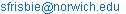(S. H. Frisbie)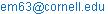(E. J. Mitchell)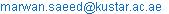(M. S. Abualrub)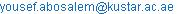(Y. Abosalem)

Seth H. Frisbie, Erika J. Mitchell, Marwan S. Abualrub, Yousef Abosalem. Calculating the Lowest Reportable Concentrations of Toxic Chemicals in the Environment. International Journal of Applied Mathematics and Theoretical Physics. Vol. 1, No. 1, 2015, pp. 9-13. doi: 10.11648/j.ijamtp.20150101.12

Abstract: The equation promulgated by 40 Code of Federal Regulations (CFR) to calculate method detection limit (MDL) and used since 1981 in the United States and other countries to protect public health and the environment is incorrect. As a result, toxic chemicals in a large number of air, food, water, wastes, and other environmental samples may in fact be present at measurable concentrations even though they are currently being reported as "not detected". That is, the air we breathe, the food we eat, and the water and other liquids we drink may have measurable concentrations of toxic chemicals, despite being reported as not detected and assumed to be absent. Furthermore, many chemicals, such as arsenic (As) in drinking water, are so toxic that they cannot be measured to safe levels and the allowable limits must be set at the lowest reportable concentrations. As a result, the allowable limits for some extremely toxic chemicals may be incorrectly set too high. Therefore, the consequences of this error pose a risk to public health and the environment. The sources of this error are explained and two improved ways of calculating method detection limit are presented.

Keywords: Method Detection Limit, Method Detection Level, Limit of Detection

1. The Definition and Calculation of Method Detection Limit

Method detection limit (MDL) is perhaps the most important statistic used for the reporting of toxic chemicals in air, drinking water, wastewater, food, hazardous wastes, and other environmental samples. A toxic chemical measured at a concentration that is less than its MDL is reported as "not detected" and is generally considered to be absent from the sample and the environment. In contrast, a toxic chemical measured at a concentration that is greater than or equal to its MDL is reported as present in the sample and the environment. MDL is used by the United States Environmental Protection Agency (U.S. EPA) to enforce the Clean Air Act (U.S. EPA 1997; U.S. EPA 2013a), Clean Water Act (U.S. EPA 1997; 40 CFR 1986), and the Resource Conservation and Recovery Act for solid wastes (U.S. EPA 1997; U.S. EPA 2013b). In contrast, the World Health Organization (WHO) does not give specific guidance for calculating the lowest reportable concentrations of toxic chemicals in drinking water (WHO 2011).

MDL is the "concentration of a substance that can be measured and reported with 99% confidence that the analyte concentration is greater than 0" (40 CFR 1986). The MDL by 40 CFR is calculated as follows. Typically, 7 aliquots of the most dilute standard solution used for calibration are prepared. This dilute standard is not a blank; that is, the concentration of analyte is greater than 0. These 7 aliquots are analyzed as samples using all of the processing steps that are specified by the method. Therefore, the number of samples (n) = 7. The results from these 7 analyses are used to calculate a sample standard deviation (s). The critical 1-tailed Student’s t value at 99% confidence and n - 1 = 6 degrees of freedom is 3.14. The product of 3.14 times s is the MDL by 40 CFR for n = 7 (see Equation 1).

(Equation 1) MDL by 40 CFR = 3.14s

Finally, the MDL by 40 CFR is compared to the actual analyte concentration of the standard solution used in this experiment. This analyte concentration must be 1 to 5 times the MDL by 40 CFR; otherwise, the experiment must be repeated using a different standard until this criterion is satisfied (APHA et al. 2005; Glaser et al. 1981).

An example of this calculation for the determination of arsenic (As) in drinking water by the arsenomolybdate method follows (Frisbie et al. 2005). Seven aliquots of a 14.3 µg/L standard solution were analyzed as samples using all of the processing steps that are specified by this method. The measured analyte concentration for each of these 7 samples is 18.4 µg/L, 13.6 µg/L, 13.6 µg/L, 14.2 µg/L, 16.0 µg/L, 13.6 µg/L, and 17.8 µg/L. Therefore, n = 7, s = 2.09 µg/L, and the critical 1-tailed Student’s t value at 99% confidence = 3.14. The MDL by 40 CFR = 6.57 µg/L, which is rounded up to 7 µg/L. Since the 14.3 µg/L standard solution used in this experiment is between 6.57 µg/L and 5 x 6.57 µg/L, the 7 µg/L MDL by 40 CFR is valid (see Figure 1).

2. Two Mathematical Errors that were made in the Derivation of Method Detection Limit

Unfortunately, the equation promulgated by 40 CFR to calculate MDL is incorrect and typically overestimates the True MDL by approximately 32%. The following 2 mathematical errors were made in the derivation of the MDL by 40 CFR.

The first is an algebra error that was reported by Gibbons and Coleman (2001). The sample standard deviation (s) was assumed to be a linear function of analyte concentration (C) (Glaser et al. 1981; see Equation 2).

(Equation 2) s = k0 + k1C

Where k0 is the sample standard deviation at zero analyte concentration and k1 is the slope. Then Equation 2 was simplified by setting k1 equal to 0 (Glaser et al. 1981; see Equation 3).

(Equation 3) s = k0

Finally, s was set equal to k0 /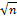(Glaser et al. 1981). This is the algebra error. Since n is an integer that is equal to or greater than 2, s cannot equal both k0 and k0 /. This causes the MDL by 40 CFR to be incorrect by a factor of 1 /.

The second mathematical error was caused by using a Student’s t distribution with the MDL set at the mean (Glaser et al. 1981; see Figure 2). That is, the analyte concentrations from -∞ to the MDL, and from the MDL to +∞ each have 50% probability (see Figure 2). It will later be shown that setting the MDL at 50% probability cutoff instead of at 99% probability cutoff causes the MDL by 40 CFR to be incorrect by a factor of 2. Keep in mind the definition of MDL by 40 CFR uses a 99% confidence level, not a 50% confidence level (40 CFR 1986).

In summary, the first error causes the MDL by 40 CFR to be incorrect by a factor of 1 /and the second error causes the MDL by 40 CFR to be incorrect by a factor of 2. Both errors cause the MDL by 40 CFR to be incorrect by a factor of 2 /(see Equation 4).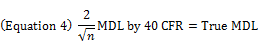Since the most common value for n is 7, the MDL by 40 CFR typically overestimates the True MDL by approximately 32% (see Equation 5).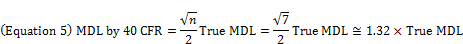3. The First Improved Method Detection Limit Based on a Two-Tailed Confidence Interval

An improved definition of MDL is based on a 2-tailed 98% confidence interval with the critical values at 0 and the MDL (see Figure 3). This True MDL does not have the 2 mathematical errors of the MDL by 40 CFR. The Student’s t distribution in Figure 1 was simply moved to the left along the x-axis until the True MDL in Figure 3 is different than 0 with 99% confidence.

Since the MDL is the concentration of analyte that is greater than 0 with 99% confidence, the concentrations of analyte from -∞ to the MDL must have 99% probability. And the concentrations of analyte from the MDL to +∞ must have 1% probability. Then by symmetry the concentrations of analyte from -∞ to 0 must also have 1% probability. Therefore, the analyte concentrations from -∞ to 0, 0 to the MDL, and the MDL to +∞ have 1%, 98%, and 1% probability, respectively. The negative analyte concentrations caused by random error are observed when the signal from a sample is less than the signal from a corresponding blank, or the y-intercept from a calibration equation.

This 2-tailed 98% confidence interval clearly shows that Equation 1 is incorrect by a factor of 2 /(see Equation 6).Where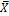= the sample mean, n = 7, and the critical 2-tailed Student’s t value at 98% confidence and n - 1 = 6 degrees of freedom = 3.14 (Neter et al. 1985; Snedecor and Cochran 1982). The critical 1-tailed Student’s t value at 99% confidence and 6 degrees of freedom, and the critical 2-tailed Student’s t value at 98% confidence and 6 degrees of freedom both = 3.14. Therefore, the analyte concentrations at 0, and the True MDL equal 0, and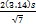, respectively (see Figure 3). That is, the True MDL is calculated as follows for n = 7 (see Equation 7).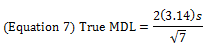Or more generally, the True MDL calculated for any n with the corresponding Student’s t value at 98% confidence and n - 1 degrees of freedom follows (see Equation 8).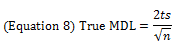Finally, when n = 7 the MDL by 40 CFR overestimates the True MDL by approximately 32% (see Equations 4 and 5, and Figure 3). This is an independent confirmation of the 2 mathematical errors made in the derivation of the MDL by 40 CFR.

Returning to the example for the determination of As in drinking water by the arsenomolybdate method, n = 7, s = 2.09 µg/L, and the critical 2-tailed Student’s t value at 98% confidence = 3.14 (Frisbie et al. 2005). Therefore, the True MDL = 4.96 µg/L, which is rounded up to 5 µg/L. The MDL by 40 CFR / the True MDL x 100% = 6.57 µg/L / 4.96 µg/L x 100%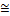132%; therefore, the MDL by 40 CFR overestimates the True MDL by approximately 32% when n = 7.

4. Two Sampling Errors that are Commonly Associated with Method Detection Limit

Furthermore, 2 sampling errors are commonly associated with the MDL by 40 CFR. An explanation of these sampling errors follows.

First, MDL by 40 CFR requires the use of standard solutions, and not blanks, to test if the analyte concentration of a sample is greater than 0. This is not logical; it uses the distribution of results at a greater concentration to estimate the distribution of results at a lesser concentration. That is, it requires that s does not change with analyte concentration. In fact, s does increase with analyte concentration for the determination of As by the arsenomolybdate, silver diethyldithiocarbamate, and graphite furnace atomic absorption spectroscopy methods (Frisbie et al. 2005). Clearly, the MDL should be based on blanks, not standard solutions. This use of standard solutions instead of blanks was never justified by the original designers of MDL (Glaser et al. 1981).

Second, MDL by 40 CFR uses the results from a single day instead of the more representative results from many days. Clearly, the MDL should be measured over many days, or even over all days that samples are analyzed, not just on 1 day.

5. Two Errors of Interpretation that are Commonly Associated with Method Detection Limit

The instructions for measuring MDL by 40 CFR are unclear (40 CFR 1986). As a result, at least 2 errors of interpretation are commonly associated with the MDL by 40 CFR. An explanation of these different interpretations follows.

First, some laboratories use 7 different standard solutions that are each analyzed 1 time, while other laboratories use only 1 standard solution that is analyzed 7 different times. This use of 7 different solutions better represents the inherent variability of a method. In contrast, this use of only 1 solution better represents the inherent variability of an instrument. Clearly, the instructions for MDL by 40 CFR should explicitly require that 7 different solutions are analyzed, not just 1 solution.

Second, some laboratories round a MDL up if the first insignificant figure is from 5 to 9 and round down if the first insignificant figure is from 0 to 4, while other laboratories always round a MDL up. This always rounding up is an attempt to error on the side of caution since it biases the MDL high approximately half the time. Clearly, the instructions for MDL by 40 CFR must clarify this ambiguity.

6. The Second Improved Method Detection Limit Based Control Charts

The final and best definition of MDL is based on the routine analysis of blanks to make a 1-tailed 99% confidence interval; therefore, the 2 mathematical errors associated with the MDL by 40 CFR are avoided. Furthermore, this MDL from Blanks uses the results from many days, or even over all days that samples are analyzed; therefore, the 2 sampling errors associated with the MDL by 40 CFR are avoided. Finally, this MDL from Blanks has consistent rules for solution preparation and rounding; therefore, the 2 errors of interpretation associated with the MDL by 40 CFR are avoided.

Most importantly, this MDL by Blanks uses the quality control data that is already collected at certified environmental testing laboratories; therefore, additional studies or costs are not required. For example, the silver diethyldithiocarbamate method for measuring As in drinking water uses 5 different concentrations of standard solutions (0 µg/L, 28.6 µg/L, 57.1 µg/L, 114 µg/L, and 229 µg/L) for the initial calibration. After the initial calibration a laboratory might analyze up to 20 samples and then test a middle standard (114 µg/L in this example) and a blank (0 µg/L) for quality control. These 114 µg/L and 0 µg/L standards are used to see if the results for the previous batch of up to 20 samples are valid. The measured concentrations of these 114 µg/L and 0 µg/L standards are compared against a running 95% confidence interval for these separate measurements. If the results for the 114 µg/L and 0 µg/L standards are within their respective 95% confidence intervals, then the results for the previous batch of sample are valid. If not, any problems must be fixed and the samples must be re-analyzed. These running confidence intervals are called control charts.

Therefore, it is logical to use the results from 0 concentration control charts to calculate a method detection limit that is based on a 1-tailed 99% confidence interval. Laboratories already have this data, so it’s readily available without any additional cost. In addition, it’s based on many days of results, not just on 1 day.

7. Conclusions

The equation promulgated by 40 CFR in 1984 and still used today to calculate MDL is incorrect and typically overestimates the True MDL by approximately 32% (see Figure 3). Therefore, toxic chemicals in a large number of air, drinking water, wastewater, food, hazardous wastes, and other environmental samples may in fact be present at measurable concentrations even though they are currently being reported as "not detected". In addition, the allowable limits for some extremely toxic chemicals may be incorrectly set too high. The consequences of this error pose a risk to public health and the environment. Therefore, it is crucial for this law to be amended so that the MDL by 40 CFR (see Equation 1) is replaced with the True MDL (see Equations 7 and 8), or an MDL by Blanks that is based control charts. Of these 2 solutions, an MDL by Blanks is preferred over the True MDL.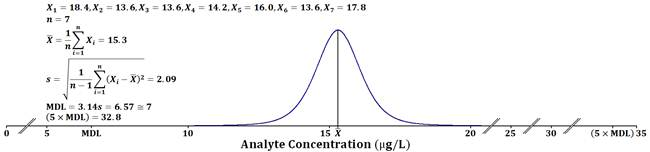Figure 1. The data and equations used to calculate MDL by 40 CFR for the arsenomolybdate method (Frisbie et al. 2005; Glaser et al. 1981). The analyte concentrations = X1, X2, …, and X7, the number of samples = n, the sample mean =, and the sample standard deviation = s.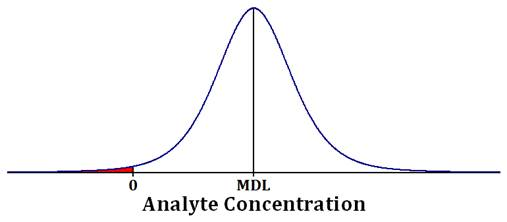Figure 2. The Student’s t distribution used to calculate the MDL by 40 CFR (Glaser et al. 1981).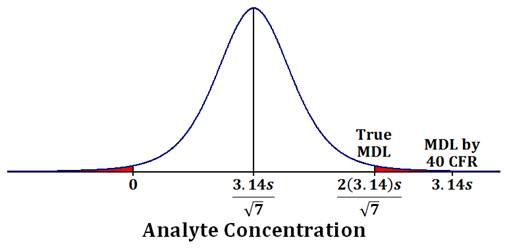Figure 3. The 2-tailed 98% confidence interval used to calculate the True MDL for n = 7. The MDL by 40 CFR overestimates the True MDL by approximately 32% when n = 7.

Acknowledgements

This study was supported by Norwich University, Better Life Laboratories, Inc., Khalifa University, and the University of Jordan. The authors are grateful to Dr. Amal Helu of the Department of Mathematics at the University of Jordan in Amman for her review of an earlier draft of this manuscript.

Abbreviation

 APHA C American Public Health Association analyte concentration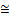approximately equals As arsenic CFR Code of Federal Regulations / divide = equals > greater than ∞ infinity < less than MDL method detection limit µg/L microgram/liter x multiply - negative n number of samples % percent ± plus minus +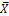k1 positive sample mean slopesquare root s sample standard deviation t Student’s t ∑ Summation U.S. EPA United States Environmental Protection Agency WHO World Health Organization k0 y-intercept

References

1. APHA (American Public Health Association), American Water Works Association, Water Environment Federation. 2005. Standard Methods for the Examination of Water and Wastewater. 21st ed. Washington, DC:American Public Health Association.
2. Frisbie SH, Mitchell EJ, Yusuf AZ, Siddiq MY, Sanchez RE, Ortega R, et al. 2005. The development and use of an innovative laboratory method for measuring arsenic in drinking water from western Bangladesh. Environ Health Perspect 113:1196-1204.
3. Gibbons RD, Coleman DE. 2001. Statistical Methods for Detection and Quantification of Environmental Contamination. New York:John Wiley & Sons, Inc.
4. Glaser JA, Foerst DL, McKee GD, Quave SA, Budde WL. 1981. Trace analyses for wastewaters. Environ Sci Technol 15:1426-1435.
5. Guidelines Establishing Test Procedures for the Analysis of Pollutants. 1986. 40 CFR Part 136 Appendix B.
6. Neter J, Wasserman W, Kutner MH. 1985. Applied Linear Statistical Models. 2nd ed. Homewood, IL:Irwin Press.
7. Snedecor GW, Cochran WG. 1982. Statistical Methods. 7th ed. Ames, IA:The Iowa University Press.
8. U.S. EPA (United States Environmental Protection Agency). 1997. National Environmental Laboratory Accreditation Conference: Constitution, Bylaws, and Standards. EPA 600/R-97/139. Washington, DC:U.S. Environmental Protection Agency.
9. U.S. EPA. 2013a. Quality Assurance Handbook for Air Pollution Measurement Systems, Volume II, Ambient Air Quality Monitoring Program. Washington, DC:U.S. Environmental Protection Agency.
10. U.S. EPA. 2013b. Test Methods for Evaluating Solid Wastes, Physical/Chemical Methods. EPA SW-846 [3rd ed. (Including Updates I, II, IIA, IIB, III, IIIA, IIIB, IVA and IVB]. Washington, DC:U.S. Environmental Protection Agency.
11. WHO (World Health Organization). 2011. Guidelines for Drinking-water Quality. 4th ed. Geneva, Switzerland: World Health Organization.

 Contents 1. 2. 3. 4. 5. 6. 7.
Article ToolsAbstractPDF(228K)